﻿ CT系统参数标定及成像研究 Study on Parameters Calibration and Imaging of CT System

Vol. 08  No. 02 ( 2019 ), Article ID: 28907 , 12 pages
10.12677/AAM.2019.82031

Study on Parameters Calibration and Imaging of CT System

Qian Su, Haocong Ying, Qinyi Yu, Meiling Chen, Yaqun Huang, Murong Jiang

School of Information Science & Engineering, Yunnan University, Kunming Yunnan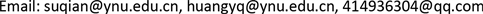Received: Jan. 27th, 2019; accepted: Feb. 11th, 2019; published: Feb. 19th, 2019ABSTRACT

The errors arising during installation of CT system will affect the imaging quality of the samples to some extent. In this paper, a method is proposed to image the unknown structure samples by calibrating the parameters of CT system with the known structure template. Firstly, based on convolution inverse projection method for image reconstruction, we discuss the main parameters calibration of a typical two-dimensional CT system with the help of the known structure template and its X-ray receiving information. Then, based on the obtained calibration parameters, a model for calculating the absorptivity based on image gray level is established to study the imaging problem of unknown structure samples. Finally, the error factors affecting accuracy and stability of the calibration are analyzed, and a new template is designed to improve accuracy and stability. The research in this paper has a certain significance to reduce the influence of CT system installation error and improve the imaging quality of the sample.

Keywords:CT System, Parameters Calibration, Convolution Method, Image Grayscale, Image Reconstruction

CT系统参数标定及成像研究CT系统安装时存在的误差在一定程度上影响到样品的成像质量，本文提出了一种借助已知结构模板对CT系统进行参数标定后再对未知结构样品进行成像的方法。首先，借助已知结构的模板及其X射线接收信息，基于卷积逆投影图像重建方法讨论了一种已安装好的典型二维CT系统的主要参数标定；然后利用已获得的标定参数，建立基于图像灰度的吸收率计算模型，研究未知结构的样品成像问题；最后对影响标定精度和稳定性的误差因素进行分析，设计新模板以改进标定精度和稳定性。本文的研究对降低CT系统安装误差影响、提高样品成像质量具有一定意义。1. 引言

2. CT系统主要参数标定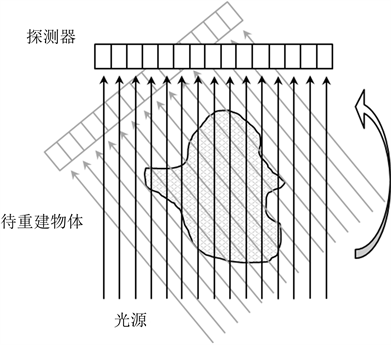Figure 1. Two-dimensional CT system schematic diagram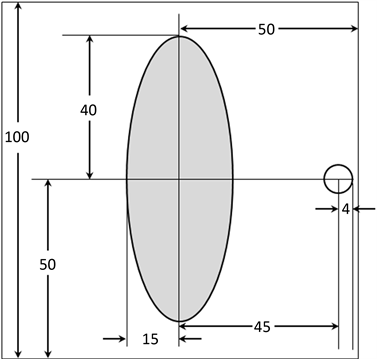Figure 2. Geometric information of known structural templates

2.1. 探测器单元间距的计算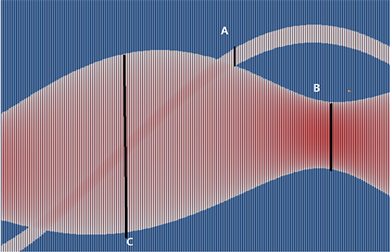Figure 3. Absorption chromatogram

${n}_{A}\approx 29$${l}_{A}=8\text{\hspace{0.17em}}\text{mm}$

$△{d}_{A}=\frac{8}{29}\text{\hspace{0.17em}}\text{mm}\approx 0.2759\text{\hspace{0.17em}}\text{mm}$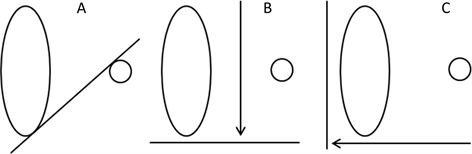Figure 4. X-ray scanning at three positions

${n}_{B}\approx 109$${l}_{B}=30\text{\hspace{0.17em}}\text{mm}$

$△{d}_{B}=\frac{30}{109}\text{\hspace{0.17em}}\text{mm}\approx 0.2752\text{\hspace{0.17em}}\text{mm}$

${n}_{C}\approx 289$${l}_{C}=80\text{\hspace{0.17em}}\text{mm}$

$△{d}_{C}=\frac{80}{289}\text{\hspace{0.17em}}\text{mm}\approx 0.2768\text{\hspace{0.17em}}\text{mm}$

2.2. 卷积逆投影图像重建

$F\left(u,v\right)={\int }_{-\infty }^{\infty }{\int }_{-\infty }^{\infty }f\left(x,y\right){\text{e}}^{-j2\text{π}\left(ux+vy\right)}\text{d}x\text{d}y$

$f\left(x,y\right)={\int }_{-\infty }^{\infty }{\int }_{-\infty }^{\infty }F\left(u,v\right){\text{e}}^{j2\text{π}\left(ux+vy\right)}\text{d}u\text{d}y$ (1)

$u=r\mathrm{cos}\theta ,v=r\mathrm{sin}\theta$ 进行代换，得公式(1)的极坐标形式：

$f\left(x,y\right)={\int }_{0}^{\text{2π}}{\int }_{0}^{\infty }F\left(r,\theta \right){\text{e}}^{j2\text{π}\left(x\mathrm{cos}\theta +y\mathrm{sin}\theta \right)}r\text{d}r\text{d}\theta$

$f\left(x,y\right)={\int }_{0}^{\text{2π}}{\int }_{0}^{\infty }F\left(r,\theta \right){\text{e}}^{j2\text{π}\left(x\mathrm{cos}\theta +y\mathrm{sin}\theta \right)}|r|\text{d}r\text{d}\theta$ (2)

${f}^{\prime }\left(x,y,\theta \right)={\int }_{-\infty }^{\infty }{\text{e}}^{j2\text{π}\left(x\mathrm{cos}\theta +y\mathrm{sin}\theta \right)}F\left(r,\theta \right)|r|\text{d}r$ ，公式(2)等价于：

$f\left(x,y\right)={\int }_{0}^{\text{π}}{f}^{\prime }\left(x,y,\theta \right)\text{d}\theta$ (3)

$f\left(x,y\right)\approx {\int }_{0}^{\text{π}}{\int }_{\frac{-1}{2d}}^{\frac{1}{2d}}F\left(r,\theta \right){\text{e}}^{j2\text{π}\left(x\mathrm{cos}\theta +y\mathrm{sin}\theta \right)}|r|\text{d}r\text{d}\theta$

$h\left(\rho \right)={\int }_{\frac{-1}{2d}}^{\frac{1}{2d}}|r|{\text{e}}^{j2\text{π}\rho }\text{d}r$ (4)

${f}^{\prime }\left(x,y,\theta \right)={\int }_{-\infty }^{\infty }g\left(\rho ,\theta \right)h\left(x\mathrm{cos}\theta +y\mathrm{sin}\theta -\rho \right)\text{d}\rho$ (5)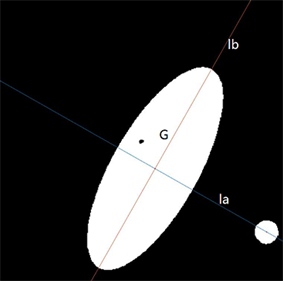Figure 5. Restoring image to establish coordinate system

la是经过椭圆心、圆心的直线，lb为椭圆长轴。原图中，椭圆长短轴的交点、圆心的位置是已知的，计算两线的方程为：

$\text{la}:y=-0.5677x+258.0935$

$\text{lb}:y=1.7613x-204.6182$

2.3. 平面投影角度计算模型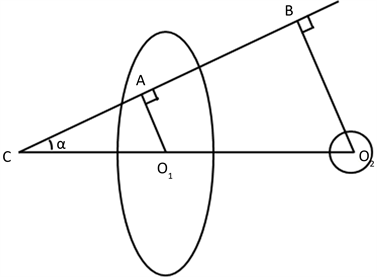Figure 6. Centroid distance projection model

|O1O2|已知，AO1、BO2分别经过椭圆心、圆心，由前述线性相关性，得出这两条X射线应是穿过椭圆和圆的射线中接收强度最大的两条，因此通过筛选出文献  附件2中每列经过椭圆的射线接收强度最大值和经过圆的射线接收强度最大值，就可找到每次旋转后的AO1和BO2，两线间距离为投影|AB|，从而可得 的值。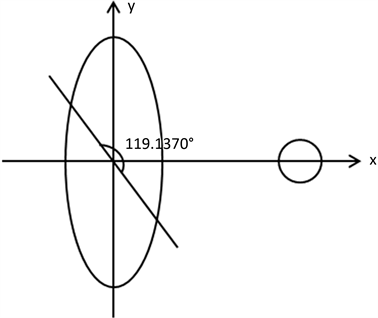Figure 7. Initial direction of X-ray

3. 未知结构样品成像研究

3.1. 图像的重建和位置还原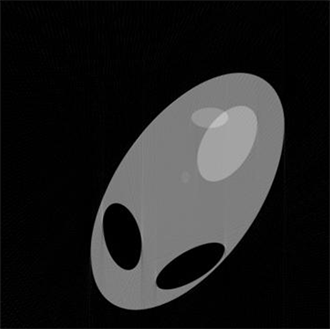Figure 8. Shape reconstruction of sample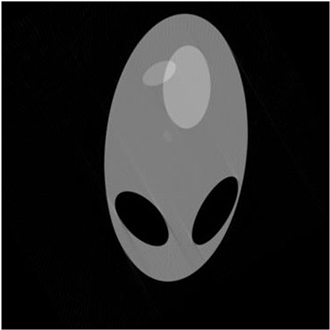Figure 9. Position of sample on pallet after cutting, rotating and translating

3.2. 基于图像灰度的吸收率计算模型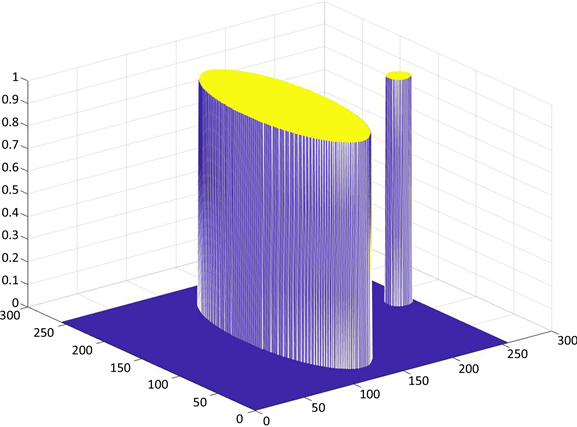Figure 10. Absorption chromatogram of annex 1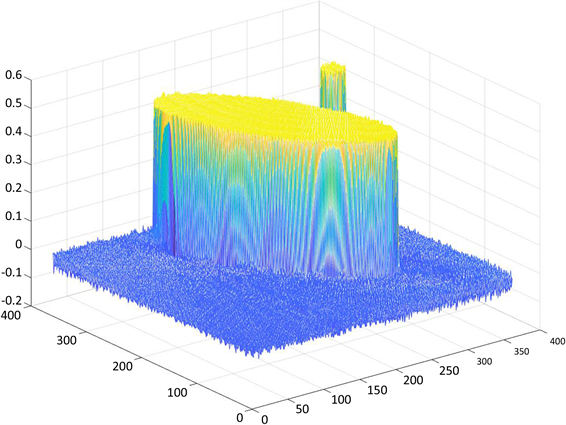Figure 11. Gray matrix after image reconstruction of annex 2

$\left\{\begin{array}{l}{\alpha }_{\left(i,j\right)}=\delta \ast {g}_{\left(i,j\right)}\\ \delta =1/{\left[{g}_{\left(i,j\right)}\right]}_{\mathrm{max}}\end{array}$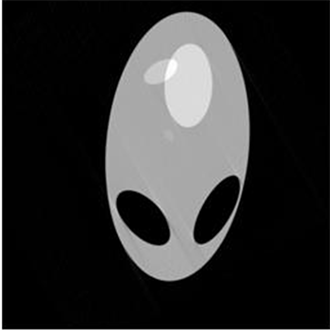Figure 12. Standardized gray image

4. 标定精度及稳定性

4.1. 影响因素分析

1) 标定探测器相邻单元之间距离时的误差。

2) 计算探测器各个时刻位置时的误差

3) 确定重建图像和原图之间关系时的误差

4.2. 新模板设计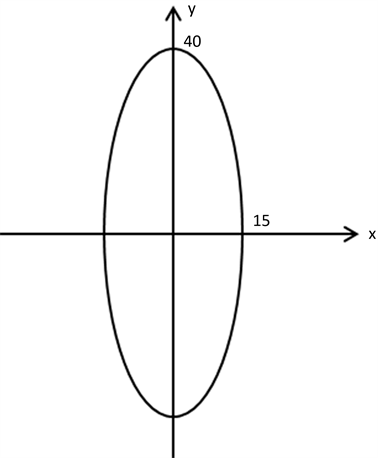Figure 13. Template for first calibration

5. 结束语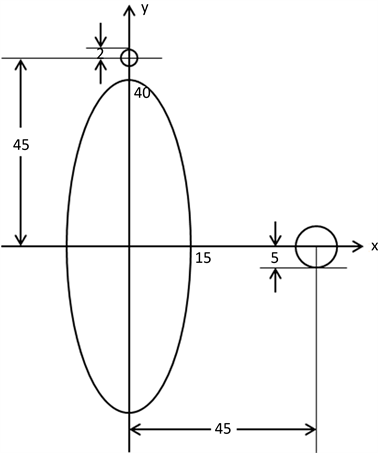Figure 14. Template for second calibration

Study on Parameters Calibration and Imaging of CT System[J]. 应用数学进展, 2019, 08(02): 265-276. https://doi.org/10.12677/AAM.2019.82031

1. 1. 科普中国. CT (电子计算机断层扫描) [EB/OL]. https://baike.baidu.com/item/CT/122415?fr=aladdin, 2017-03-20.

2. 2. 余晓锷, 龚剑. CT原理与技术[M]. 北京: 科学出版社, 2014.

3. 3. 全国大学生数学建模竞赛组委会. 2017年高教社杯全国大学生数学建模竞赛赛题[EB/OL]. http://www.mcm.edu.cn/html_cn/node/460baf68ab0ed0e1e557a0c79b1c4648.html, 2017-09-14.

4. 4. 闫镔, 李磊. CT图像重建算法[M]. 北京: 科学出版社, 2014.

5. 5. 伍伟文, 全超, 刘丰林. 相对平行直线扫描CT滤波反投影图像重建[J]. 光学学报, 2016, 36(9): 157-167.

6. 6. Jiang Hsieh. 计算机断层成像技术处理原理、设计、伪像和进展[M]. 北京: 科学出版社, 2006.

7. 7. 张顺利. ART算法几种重建模型的研究和比较[J]. 航空计算技术, 2005, 35(2): 39-41.

8. 8. 王会鹏, 周利莉, 张杰. 一种基于区域的双三次图像插值算法[J]. 计算机工程, 2010, 36(19): 216-218.